# Variables and Data Types in VBA Excel

• Difficulty Level : Expert
• Last Updated : 31 Mar, 2022

In a computer system, variables and data types are almost used in every program to store and represent data. Similarly, Excel VBA also has variables and data types to store and represent data and its type. In this article, we will learn about VBA variables, their scope, data types, and much more.

### VBA Variables

VBA(Visual Basic for Application) variables are similar to other programming languages variables, they act as a container that is used to store data(integer, string, floats, etc). We can use the variables in the code at multiple places and executer the programs.

Defining Variables In VBA

VBA gives permission to define variables in two ways:

1. Implicitly – In VBA, we can implicitly declare variables using the assignment(=) operator. All the variables that are implicitly declared in VBA are of type “Variant“. The variant type variables required more memory space than usual variables. Example: label=”gfg”
2. Explicitly – Explicitly we can declare variables using “Dim” keyword. Explicit variable also reduces the naming conflicts and spelling mistakes. Example: Num as password

Syntax For VBA Variables

// macro definition

Sub VBA_Variable_Example ()

Dim <name>

End Sub

VBA Variables Rule:

1. Its length should be less than 255 characters.
2. No space is allowed between characters.
3. It should not starts with an integer.
4. Period(.) is not allowed in between the characters.

Example:

In this example, we will define a macro in excel, And we will enter the code inside that macro and execute the code to understand the working of VBA variables in excel.

Step 1: First, we will make our Developer option available in the Excel toolbar. For this, go to any of the tools(here, we are choosing Draw) and then right-click on it and select the “Customize the Ribbon..” option.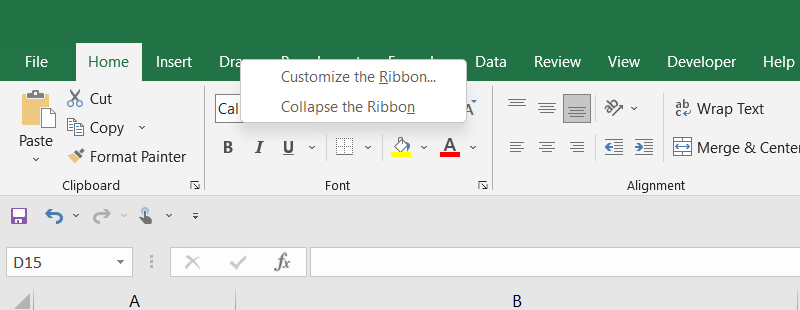The excel will open pop-up options, there we need to check the Developer checkbox and click on OK. This will enable the Developer option and make it available in the excel top toolbar.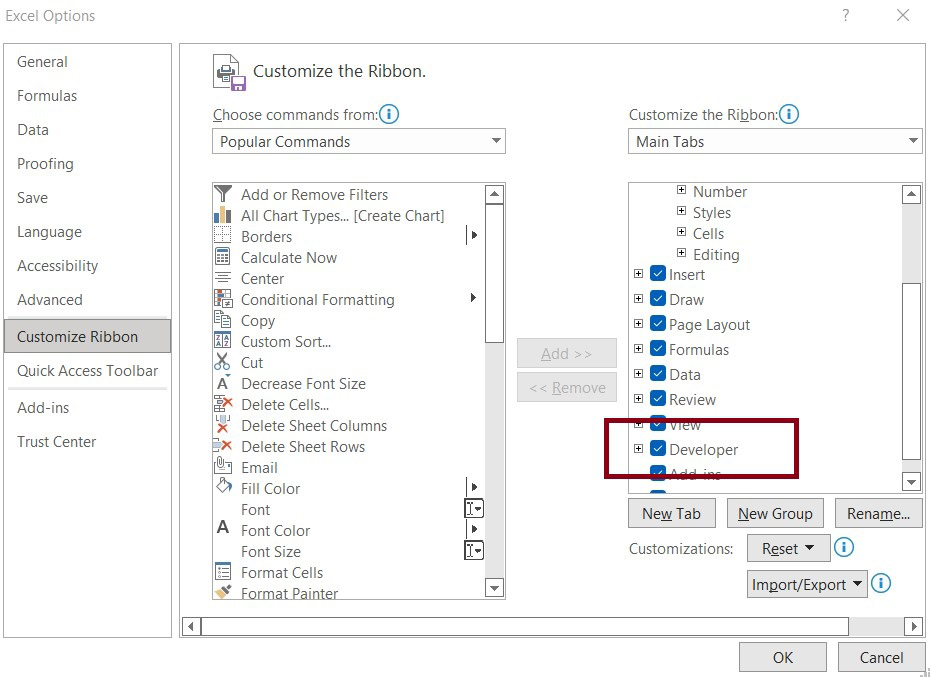Step 2: In this step, we will declare our variable in the visual basic editor. For this go to Developer > Visual Basic Editor.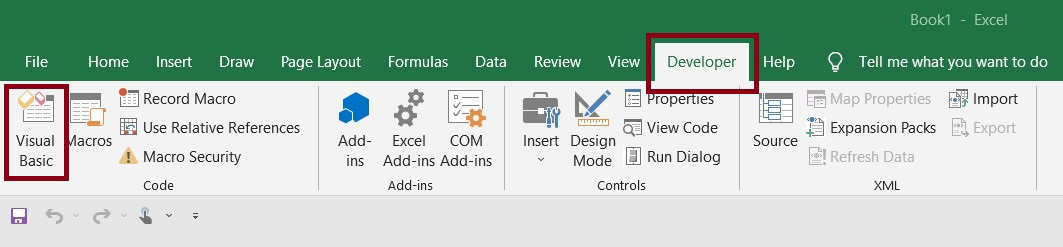This will open the Visual Basic Code Editor, where we are required to write our VBA script.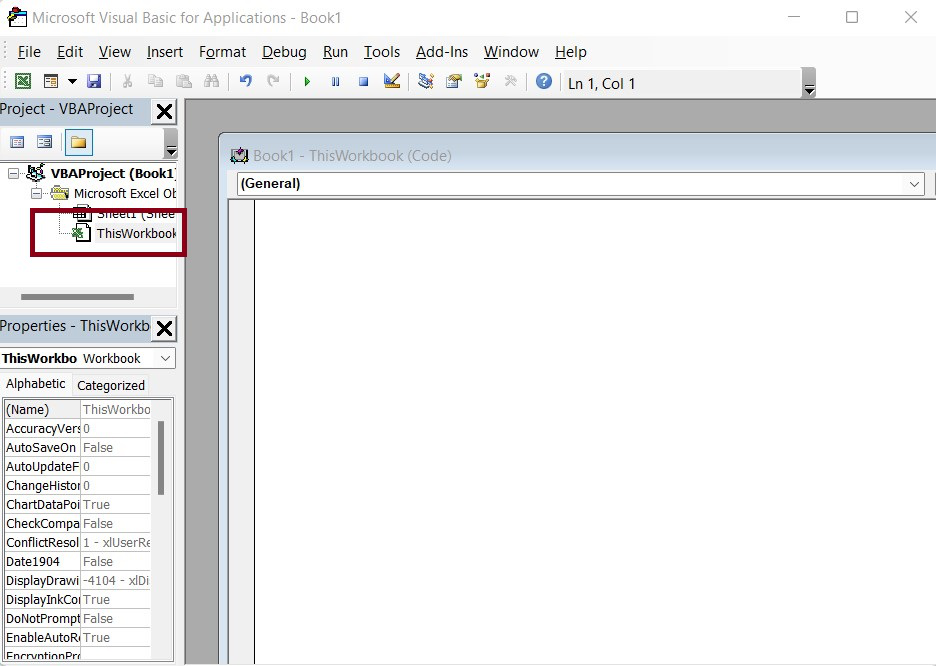Step 3: In this step, we will write our VBA scripts. For this, we will double click on ThisWorkbook under Microsoft excel objects in the left pan and open the editor and write the following code to it.

Sub VBA_Variable_GFG_Example()

Range(“a1”).Value = “Data Structure Course”

Range(“b1”).Value = “Data Structure Course”

Range(“c1”).Value = “Data Structure Course”

Var = “Data Structure Course”

Range(“a3”).Value = Var

Range(“b3”).Value = Var

Range(“c3”).Value = Var

End Sub

In the above, we can see without using variables if we want to make changes to the string “Data Structure Course” and add “GeeksForGeeks” before every string, we need to repeat it at three different places. But if we use a variable then we just need to change it at one place where we declare our variable. This will reduce the workload.

Sub VBA_Variable_GFG_Example()

Range(“a1”).Value = “Data Structure Course”

Range(“b1”).Value = “Data Structure Course”

Range(“c1”).Value = “Data Structure Course”

Var = “GeeksForGeeks – Data Structure Course”

Range(“a3”).Value = Var

Range(“b3”).Value = Var

Range(“c3”).Value = Var

End Sub

We will write this code in the VBS script editor and execute it. This will print the output string in the cells defined in the code.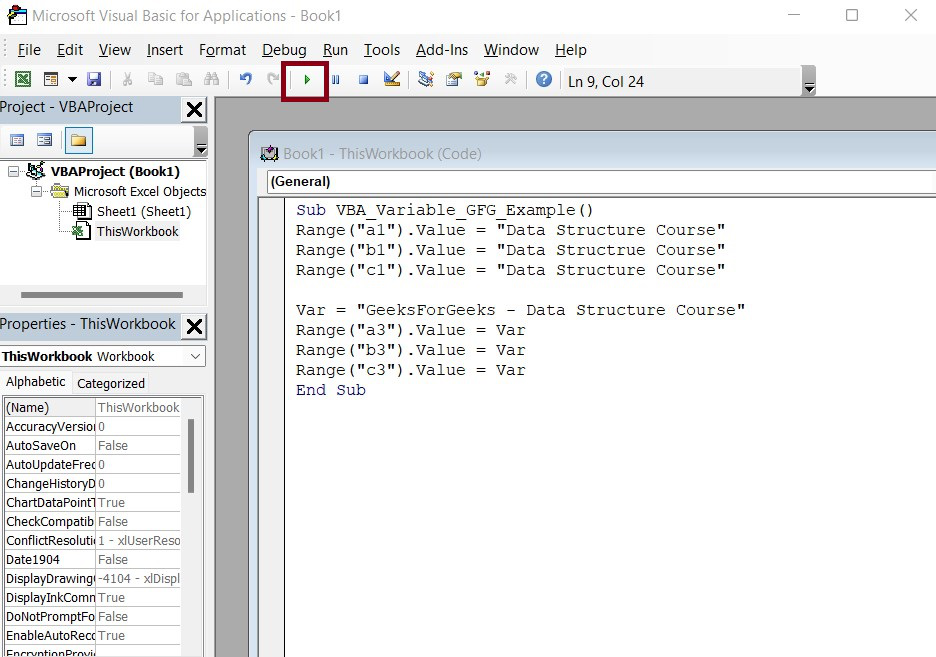Once, we execute it using the Run button, we will get the following output.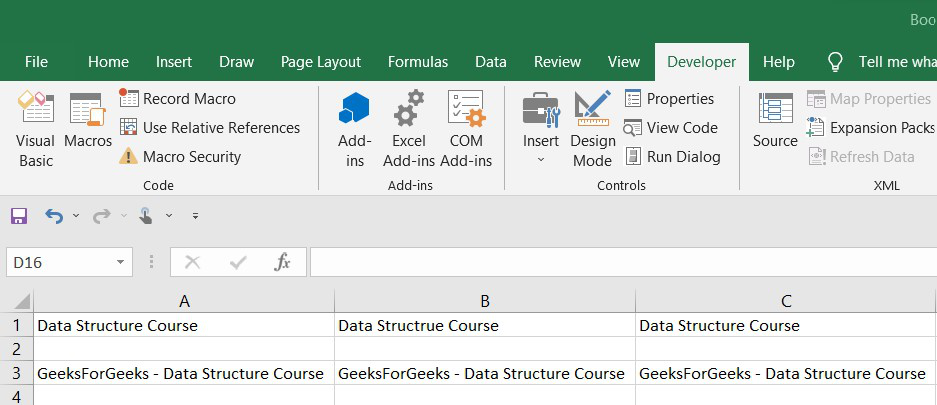### Scope Of VBA Variables

The scope of variables is determined when we declare the variable. In VBA, the scope tells where the variable may be used. There is three level of scopes of the variable:

1. Procedure Level: These are those variables that can be used within the procedure they are defined. A Procedure is either a Sub or a Function

Example: In this example, we will see the procedure level of the VBA variable’s scope. For this open the VBA editor and write the following code to it.

Sub sub1()

Dim res As String

res = “The variable which can be used within the procedure they are defined in.”

Range(“a1”).Value = res

End Sub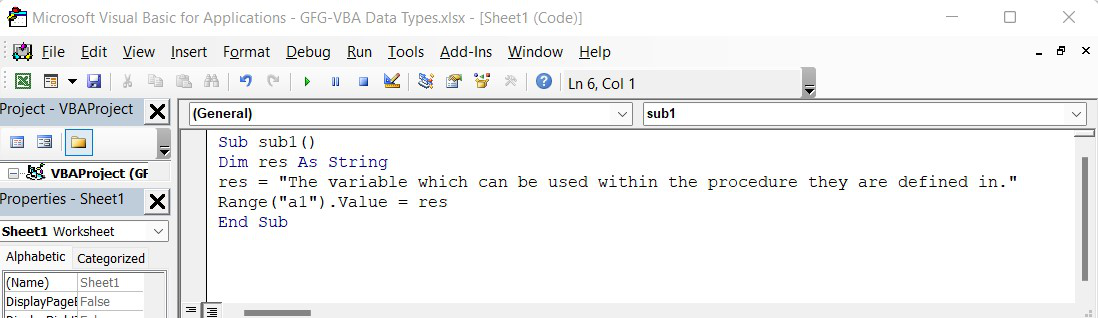Once, we click on the Run button in the VBA editor, we will the output. The text will get printed to cell A1.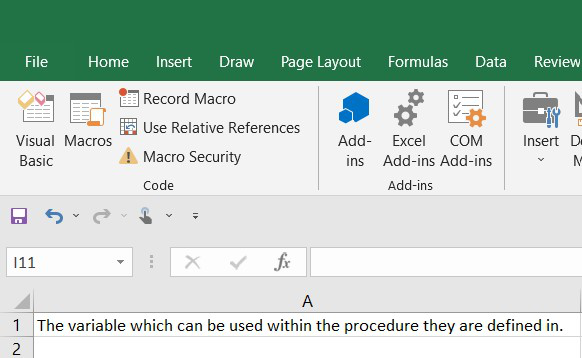2. Module Level: It is also known as the Private Module Level. It can be used by any procedures within the same module. These variables must be declared outside of all the procedures, at the top of the module.

Example: In this example, we will look for the module scope of variables. For this, we will create a new module. We will open VBA Editor and in the left pane (project explorer) we will Right-Click and create a new module add the following code to it.

Dim txt As String

Sub ProcedureDemo()

txt = “A Sub or Function is a Procedure, variables defined inside them only accessible within them”

Range(“a1”).Value = txt

End Sub

Sub PrivateModuleDemo()

txt = “Variable which is define inside a module, are accessible by all the procedures within that module”

Range(“a2”).Value = txt

End Sub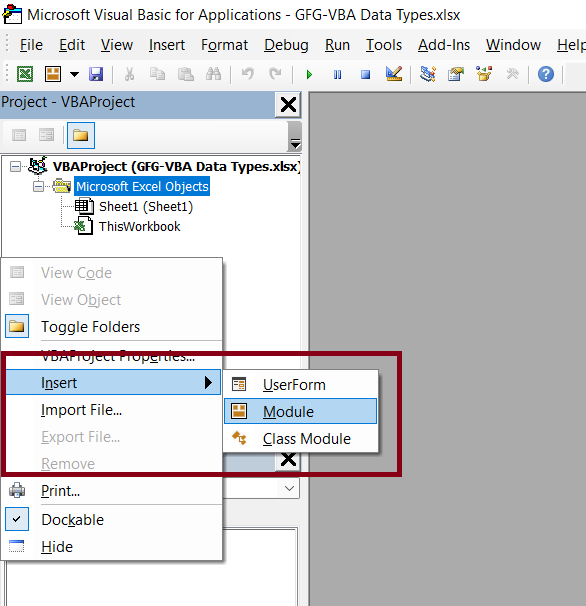After this, we just need to click on the Run button. Also, when we run it, it asks for which macros to run, there we need to choose “Macros In: <All Projects>“.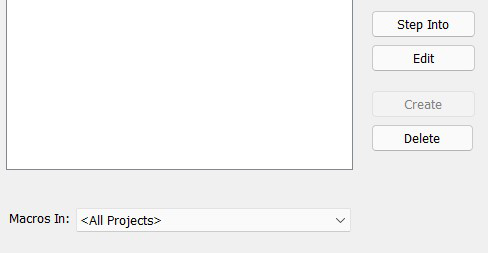Once we run it, we will get the output in cells A1 and A2.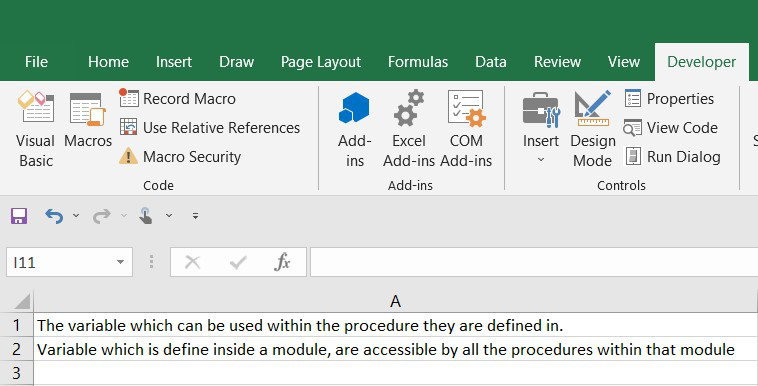3. Public Module Level: As the name suggests, the public module variables scope is at the Project level. If we define any variable as a public module variable it can be used in any module inside that project.

Example: In this example, we will add two different modules and define a public VBA variable inside one module and try to access it from both modules. Below is the code for module1 and module2.

Module1

Public res As String

Sub demo_1()

res = “geeks”

Range(“a1”).Value = res

End Sub

Module2

Sub demo_2()

res = “geeksforgeeks”

Range(“a2”).Value = res

End Sub

Once, done with both the modules. Just click on the Run button. The output will get printed in cells A1 and A2,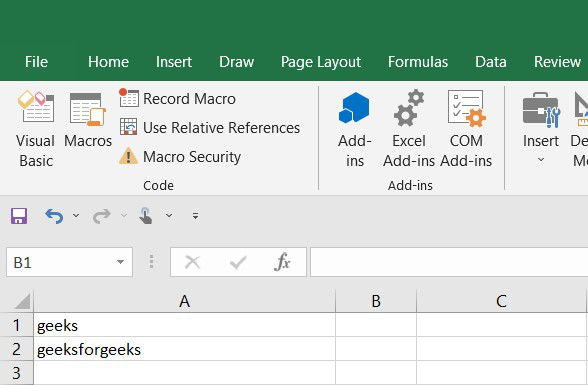Fig 12 – Output

The variables can retain their value up to their scope. The lifetime of a variable tells about how long a variable can retain its value. In order to extend the scope and so, the lifetime of a variable we need to declare the variable with a static keyword. If we declare a variable with a static keyword, the variables will retain their value even after all the macros are finished execution.

Example: In this example, we will define a variable using the static keyword and execute it multiple time to check whether it retains the value or not. We need to write the following code to the VBA Editor.

Sub StaticVariableDemo()

Static count As Integer

count = count + 1

MsgBox (count)

End Sub

After this, we need to execute it. Every time we will click on the run button the MessageBox will return the updated value. (1, 2, 3…..) and thus it will extend its scope and lifetime.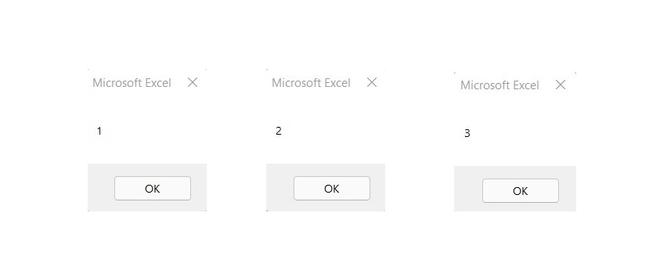### VBA Data Types

In computers, we use data types to differentiate between the integers and strings, etc. The data types which we will assign to a variable, decide what should be stored in that variable, i.e., the values that need to be stored in the variable is depends on the data type of the variable. In VBA, data types are divided into two major types:

1. Numeric Data Type: Numeric data types are used to perform mathematical operations such as addition, subtraction, etc. It is used to handle the numbers in various representations format. In numeric data type, the Integral Type represents only the whole numbers(zero, positive & negative). Non-Integral Types represent both the integer and the fractional part.

2. Non-Numeric Data Type: Non-Numeric data types are not manipulated by the arithmetic operators. These are comprised of texts, data, etc.

Note: If we do not declare any data type, the VBA will be default makes variable as a variant type.

Example:

In this example, we will write a simple script to create a button and with one click of that button an event will occur and we will get the output.

Step 1: First we will insert a command button in our excel worksheet. For this go to Developer > Insert > Command Button and click on it.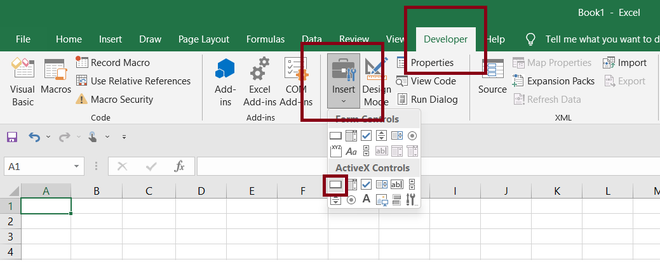Fig 14 – Command Button

After that, we can insert the button anywhere in the sheet we want. We just need to drag it over the excel sheet.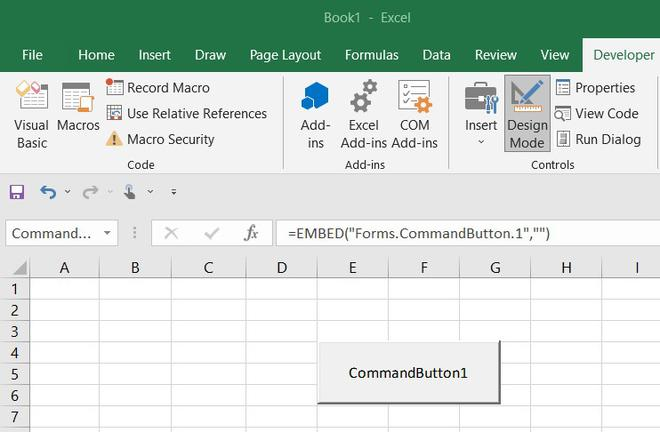Step 2: In this step, we will write the script for our button. For this just double-click on the button. This will open the VBA Script Editor where we will write the following code. In the below code, we are defining GFG and course as String data type and res as Integer data type.

Private Sub CommandButton1_Click()

Dim GFG As String, res As Integer, course As String

GFG = “GeeksForGeeks”

res = “01”

course = “Interview Preparation”

Range(“a1”).Value = GFG

Range(“b1”).Value = res

Range(“c1”).Value = course

End Sub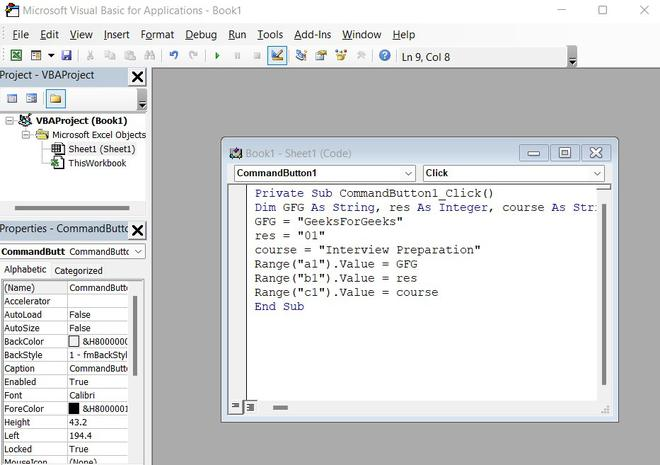Step 3: In this step, we will save our script. For this click on the Save button in the VBA Editor.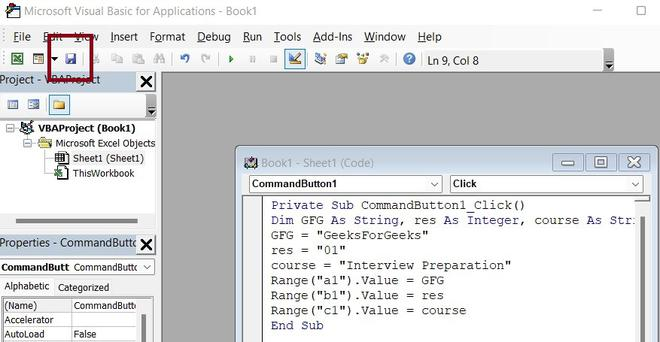The excel will popup a window asking for saving the macros. We need to click “Yes” and it will save it.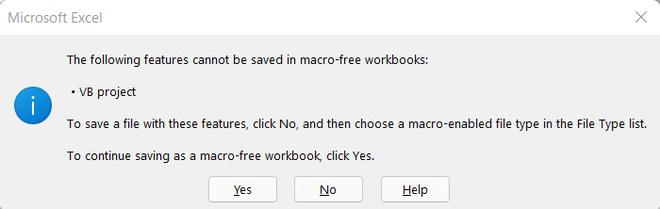Step 4: Now, we will execute our script. For this, we need to click over the command button. But before clicking over it, we are required to come out of Design Mode. To move out of any mode just click on mode once.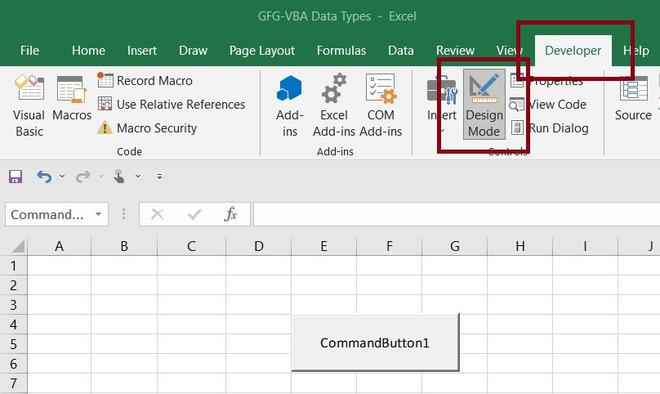Once we are done with this, we will execute our script by clicking over the commandButton1. It will give the following output.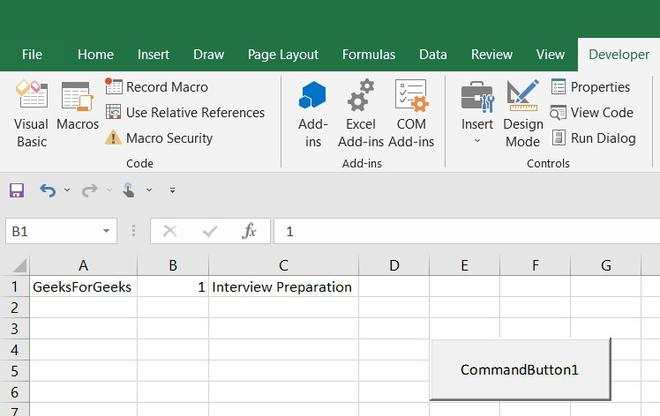Note: If we write “01” in any cell in excel, it will print “1” by default. That’s why the in output, in cell b1 only “1” is printed.

My Personal Notes arrow_drop_up
Recommended Articles
Page :